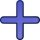Chicken

I threw three chicken for a handful of grains and I noticed that it eat in a ratio of 8:7:6 and two of them grabbed 156 grains. How many grains have fought that chicken?

Result

x =  96
y =  84
z =  72

Solution:

y+z =156
7 x = 8 y
6 y = 7 z

y+z = 156
7x-8y = 0
6y-7z = 0

x = 96
y = 84
z = 72

Calculated by our linear equations calculator.

Leave us a comment of this math problem and its solution (i.e. if it is still somewhat unclear...):Be the first to comment!To solve this verbal math problem are needed these knowledge from mathematics:

Do you have a linear equation or system of equations and looking for its solution? Or do you have quadratic equation?

Next similar math problems:

1. Family parcelsIn father will he divided the land so that the older son had three bigger part than younger son. Later elder son gave 2.5 ha field to younger and they had both the same. Determine the area of family parcel.
2. SummerjobThree students participated in the summerjob. Altogether they earn 1780, -. Peter got a third less than John and Paul got 100,- more than Peter. How much got every one of them?
3. Three workersThree workers were rewarded CZK 9200 and the money divided by the work they have done. First worker to get twice than the second, the second three times more than the third. How much money each worker received?
4. FactoryIn the factory workers work in three shifts. In the first inning operates half of all employees in the second inning and a third in the third inning 200 employees. How many employees work at the factory?
5. Unknown numberIdentify unknown number which 1/5 is 40 greater than one tenth of that number.
6. Two numbersFind two numbers whose difference and ratio is 2.
7. AlgebrogramSolve algebrogram: mama+anna=vari How many solutions are?
8. The dormitoryThe dormitory accommodates 150 pupils in 42 rooms, some of which are triple and some are quadruple. Determine how many rooms are triple and how many quadruples.
9. Spain vs USASpain lost to the US by 4 goals. In the match total fell 10 goals. How many goals gave the Spain and how the United States?
10. Waiting roomIn the waiting room are people and flies. Together they have 15 heads and 50 legs (fly has 6 legs). How many people and flies are in the waiting room?Number 118 divide into two addends, so first addend is 69 greater than 75% of the second addend.The dining room has 11 tables (six and eight seats). In total there are 78 seats in the dining room. How many are six-and eight-seat tables?Mother is 39 years old. Her daughter is 15 years. For many years will mother be four times older than the daughter?Theatrical performance was attended by 480 spectators. Women were in the audience 40 more than men and children 60 less than half of adult spectators. How many men, women and children attended a theater performance?We have two numbers. Their sum is 140. One-fifth of the first number is equal to half the second number. Determine those unknown numbers.In a football match with the Italy lost 3 goals with Germans. Totally fell 5 goals in the match. Determine the number of goals of Italy and Germany.Dalibor and Adam together scored 97 goals in the season. Adam scored 9 goals more than Dalibor. How many goals scored each?